Next: Example of Calorimeter Uncertainties Up: W Mass by Direct Previous: Selecting WW Events

## Jets, Leptons and Kinematic Fitting

In a selected W-pair event with two or four jets, the reconstructed W mass is obtained from the di-jet mass. One sees, assuming the simple case of massless jets, that the di-jet mass mJ1J2 depends on the jet energies and angles;

mJ1J22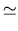2EJ1EJ2(1 - cos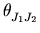).

Jets are obtained by clustering detector objects with a chosen algorithm. The hadronic channel poses particular problems arising from mis-assignment of soft particles to the wrong W, interconnection effects between particles from different W's, such as Bose-Einstein correlations and colour-reconnection, and jet combinatorics (three ways to form two di-jets from four-jet events). In the semileptonic channels, calorimeter objects created near the charged lepton, by Final State Radiation or Bremsstrahlung, can evade association to the lepton and thus degrade the event mass estimator.

Table 2 compares jet and lepton resolutions for two LEP experiments, OPAL  and ALEPH . One should note that the energy of an electron is measured much more precisely than a jet. The intrinsic energy resolution for an OPAL lead glass block is considerably better than the quoted resolution for 47 GeV electrons in OPAL implies, for a number of reasons . Predominantly this is because there are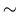2X0 of material in front of the lead glass blocks, mostly the magnet coil and pressure vessel. Smaller effects come from the reduction of the gain of the photomultipliers at LEP2, which was done to increase their dynamic range to allow for 100 GeV electrons, and the fact that the calibration of the barrel region is more difficult with a smaller sample of central detector Bhabhas.

 OPAL ALEPH Combined tracking: Combined tracking: (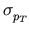/pT)2 = (0.020)2 + (0.0015pT)2/GeV2/pT = 0.0006pT + 0.005 Electromagnetic Calorimeter: Electromagnetic Calorimeter: 11704 lead glass blocks. For Barrel: Lead/wire plane sampling device/E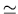0.2% + 6.3%/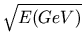/E0.9% + 18%/For Eelec47 GeV,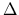E/E3%. For Eelec47 GeV,E/E3.5%. Jet Energy Resolution (Z Peak): Jet Energy Resolution (Z Peak):/E20%/E11% Jet Angular Resolution: Jet Angular Resolution: 20-30 mrad 20-30 mrad (depending on Ejet and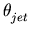) (depending on Ejet and)

The next stage of analysis is the kinematic fit. The aim of such a fit is to evaluate a set of four 4-vectors (two for each W) for each event, consistent with (a) (E, p) conservation (the LEP beam energy is known to high precision,Ebeam20 MeV for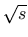= 189 GeV data); (b) Expected biases (determined from WW MC and detector simulation) e.g. average jet energy loss for a given (Ejet,); (c) Measured energies and angles of the jets and leptons, within their resolutions.

Using (E, p) conservation in the hadronic channel imposes four constraints (4C) and results in two mass values per event (for a given di-jet combination). However, an additional requirement that these two masses are equal may be imposed, resulting in a total of five constraints (5C) and only one mass value per event. This is made possible by the fact that the mass resolution of the reconstruction, which is about 2-3 GeV, is very similar to the W width,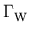. In the semileptonic channels, three constraints are lost in reconstructing the neutrino, thus 4C and 5C reduce to 1C and 2C respectively.

Kinematic fitting results in the significant improvement of mass resolution. The choice of using the additional equal mass constraint varies between LEP experiments. One can appreciate at this stage that since a tight constraint on energy is being provided by the beam energy, the most important information from jets is their direction.

For small angle jets, detector simulation predicts that the reconstructed jet energy is 30-40% lower than the true jet energy. This is not surprising, since as the polar angle decreases (towards the beamline) there are numerous subdetector boundaries and changes, and more emphasis on electromagnetic calorimetry for luminosity measurements. The expected energy loss is implemented in the kinematic fit, however one must ensure it correctly reproduces the effect in data. This is achieved by looking at Z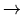q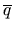events at the Z-pole, noting that in a two-jet event, the true jet energy should be the same value as the precisely known beam energy. Thus any discrepency in energy loss between data and Monte Carlo can be accounted for, and for the LEP experiments it is typically 2-3% in the most forward regions. DELPHI additionally use 3-jet events . ALEPH correct for the effect, using the error on the correction as a systematic error .

Three of the four LEP experiments use a Monte Carlo reweighting method [4,9,10]. A large sample of Monte Carlo events generated with a known mW are reweighted to different mW values using matrix element information, until the MC mass distributions best fit the data. If the MC correctly models the data, biases are implicitly accounted for. ISR is implemented in the MC up to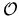(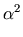L2) and a systematic error can be estimated to represent the omission of higher order terms. DELPHI employ a convolution method .Next: Example of Calorimeter Uncertainties Up: W Mass by Direct Previous: Selecting WW Events
latex2html conversion by www person on 1999-12-05